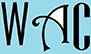## Algebra Question for Title 1 Schools: How Long Question?

2) If Anne can paint a room in 4 hours and Peter can do the same in 6 hours, how long will it take both of them working together?

distance = rate x time

rate = distance / time

1/4 + 1/6 = 1/x (we add because they are working together; the room is the numerator and their hours denominator)

The LCM of 4 (2 x2) and 6 (2×3) = 12 because you take the longest chain of each number (2x2x3 = 12).  Then you divide the LCM by each denominator and multiple each  answer with their respective numerator.

(3 + 2)/12 = 1/x

5/12 = 1/x

you have to make “x” subject of formula by moving “x” to the other side to make it a numerator. When you go to the other side, addition becomes subtraction, multiplication becomes division, and vice versa.

x/1 = 12/5

x = 12/5 = 2 2/5

2/5 of 60 minutes = 24 minutes

x = 2 hours 24 minutes for both Anna and Peter to paint the room together.